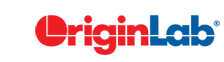# 5.5 Use X-Function in LabTalk

## Summary

X-Functions provide a uniform way to access many of Origin's capabilities from your LabTalk scripts.

The syntax to call an X-Function is:

xFunctionName [argument1:=<range,name or value> argument2:=<range,name or value> ... ][ -switch];

Option switches allow you to access alternate modes of executing X-functions from your scripts. For each X-Function, you can run the -h switch to access its help file to output arguments and definitions:

xFunctionName -h;

X-Function operations are often associated with an option tree, which is accessible with the op_change X-Function. It is possible to use this op_change X-Function to make changes to some arguments and re-execute the X-Function operation.

If an X-Function generates report data, you can get the data and put it to a specific worksheet/column or a tree variable.

## What You Will Learn

This tutorial will show you how to:

• call X-Function with LabTalk script
• manipulate tree node options for X-Function
• generate script from dialog settings
• get the operation tree, change settings and run X-Function again with new settings
• send result data to tree variable or worksheet/column
• use switch options when accessing X-Function

## Steps

### Call X-Function

1. Launch Origin and start with a new empty OPJ file. Call the X-Function newbook to create a new workbook.
2. //The workbook long name will be "Curves"
//The workbook short name will be stored in string variable bkn$newbook name:="Curves" result:=bkn$;
3. Run the following script to open the graphical interface for impASC x function, i.e. the Import ASCII dialog:
4. impASC -d;
//"-d" is an execution option of x function, which is used to open the graphical interface
5. Browse to select the file Polynomial Fit.dat under <Origin Exe Folder>\Samples\Curve Fitting\ path
6. Under the Import Options tree node, select No for Add Sparklines, and uncheck Rename Sheet with (Partial) Filename, Rename Book with (Partial) Filename and Append Filename to Workbook Comment.
7. Click the triangle button next to the Dialog Theme, choose Generate Script in the fly-out menu, the LabTalk script for this operation will be typed to the script window.
8. Click Cancel to exit the ImpASC dialog, and the script should be dumped in Script Window. We rewrite it into multiple line so that you can read it easily.
9. //Note: the file path may differ from your Origin EXE path

impASC fname:="C:\Program Files\OriginLab\Origin2016\Samples\Curve Fitting\Polynomial Fit.dat"
options.sparklines:=0
options.FileStruct.NumericSeparator:=0
options.Cols.ColDesign:=<Unchanged>
options.Names.FNameToSht:=0
options.Names.FNameToBk:=0
options.Names.FNameToBkComm:=0;
10. If it's a online script. Just put cursor on the line and press Enter to execute it. If it's in multiple lines, highlight them and press Enter to run it. The "options" in the script above is a tree variable, which is used to set and store parameters for this X-Function.
11. Use the fitpoly X-Function to carry out polynomial fit for the XY dataset in the first two columns, use the "-r" switch option to set the recalculation mode to "Auto".
12. fitpoly iy:=[%(bkn$)]!(1,2) polyorder:=2 coef:=<new> oy:=[<Input>]<new>!<new> AdjRSq:=arsq RSqCOD:=rsq -r 1; ### Recalculate of X-Function 1. Now get a range variable that contains this operation, which will be used later. 2. range r1 = [%(bkn$)]%(page.active$)!col(1); 3. Now get the setting in the operation as the tree variable mytree. 4. op_change ir:=r1 tr:=mytree; 5. In Script Window, run the following script to get the tree structure and values. 6. mytree.=; 7. You can now change operation settings using the mytree variable. 8. //Change the input data mytree.xfGetN.iy.Range1.Y$ = "[%(bkn$)]1!C"; //Change the polynomial order mytree.xfGetN.polyorder = 3; 9. You can run the script "mytree.=;" again to check the modified settings. 10. Now execute the operation again with the modified settings. 11. op_change ir:=r1 tr:=mytree op:=run; ### Get Results from X-Functions You can get report data from an X-Function operation and send it to a specific book/sheet/column location, or a tree variable. 1. Create a new worksheet with the newsheet X-Function. 2. newsheet; //Get the sheet name in string register %B %B = page.active$;
3. Fill in some data in column A.
4. col(A) = {13.2, 14.1, 11.7, 23.9, 10.3};
5. Use the grubbs X-Function to detect if there is an outlier in column A.
6. //Output the report data into tree variable tr
//If tr does not exist yet, it will be created
grubbs ix:=col(A) rt:=tr;
7. Use the following script to get the tree structure and values.
8. tr.=;
9. Get result values from tree variable and pass to double or string variables.
10. //Get the row index for suspected outlier
double rindex = tr.Stats.Stats.C2;
//Get the conclusion string
string conclusion$= tr.Stats.Footer$;
//Display the conclusion sentence right to the data cell of the suspected outlier
col(B)[$(rindex)]$ = conclusion\$;
//Change column width to display all texts
wks.col2.width=40;
11. Run the grubbs x function again, but send the report data to a specified worksheet Result.
12. //Create the new worksheet "Result"
newsheet name:=Result;
//Send the report data to the Result worksheet
grubbs ix:=[%H]%B!col(A) rt:=[<Input>]Result!;
//Note: the above newsheet script isn't necessary since if sheet with name Result doesn't exist,
//it will be created automatically.To get report tree of nonlinear curve fit (nlbegin/nlfit/nlend X-Functions), you may also use the X-Function getnlr.